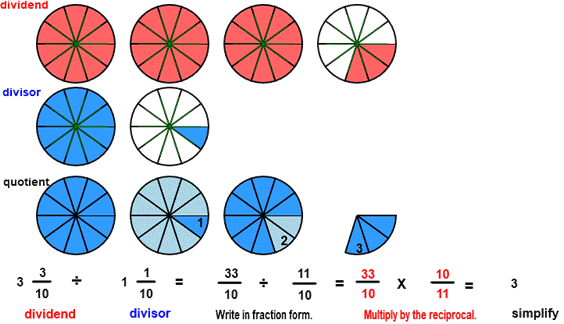DIVIDE
CORRECT:
ATTEMPTS:
SCORE:
PERCENT
WHOLE
NUMERATOR
DENOMINATOR

# DIVIDE FRACTIONS INSTRUCTIONS

Follow the directions in the dialog box after pressing the <START> button. The <EXPLAIN> button may then be pressed after you enter the divisor to see how to do the example.

The following  image was made from Divide Fractions With Circles Designe r:The parts of a division example are the dividend, the divisor, and the quotient.

When the program starts, you will be asked to identify the dividend. The picture shows a dividend of 3 310 circles.

Once the dividend is correctly identified, circles representing the divisor will appear. The picture shows a divisor of 1 110 circles. The program will not continue unless the dividend or the divisor are correctly identified.

You will then be asked to find the quotient. The quotient is the number of divisor circles that will fit into the dividend circles.

Imagine you are covering the dividend circles with the divisor circles. You might have to imagine some cutting and pasting to cover the dividend with the divisor. The third row, representing the quotient, shows how the divisor will fit into the dividend. There is a color change of light blue and dark blue after each divisor has been fit into the dividend. You can see from the image that 3 divisor circles fit into the dividend. The quotient then is 3.

All the examples in this program will have a whole number quotient from 1 to 12.

The picture shows how to calculate the quotient. First write the dividend and the divisor in fraction form as shown in the example. Then multiply 3310 by the inverse of the divisor. The inverse of the divisor is found by  replacing the numerator with the denominator and the denominator with the numerator. In short,  divide by 1110 by multiplying by 1011.

See MULTIPLY FRACTIONS  for instructions on how to multiply fractions.

See MULTIPLICATIVE INVERSE for more information on how to find the inverse.

For more instruction on dividing fractions go to How To Divide Fractions.

After you enter the quotient you may press the <REPORT> button. The report will ask for your name but you may submit a code for your name. This report will give the same results as on the dialog box. The report may be printed or e-mailed.